Monday, September 27, 2021
Home > Latest Announcement > NMTC 2019 Question Papers With Solutions Sub Junior Level Class 7 & 8

# NMTC 2019 Question Papers With Solutions Sub Junior Level Class 7 & 8# NMTC 2019 Sub Junior Level Class 7 & 8

NMTC 2019 Question Papers With Solutions Sub Junior Level Class 7 and 8 is the exam conducted by AMTI. It was scheduled on 31 August 2019 at 2 PM to 4 PM.

## NMTC 2019 Sub Junior Level Question Paper

NMTC 2019 Sub Junior Level exam was conducted on 31 August 2019.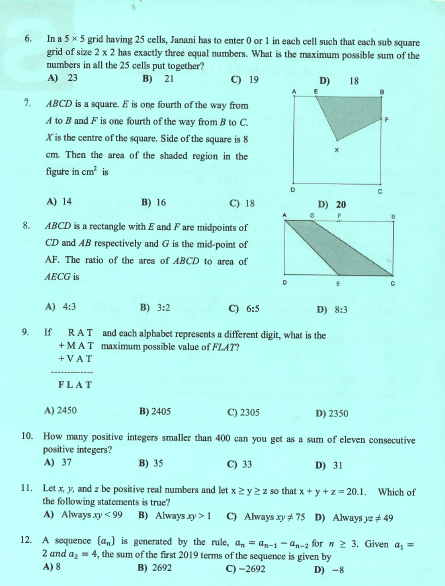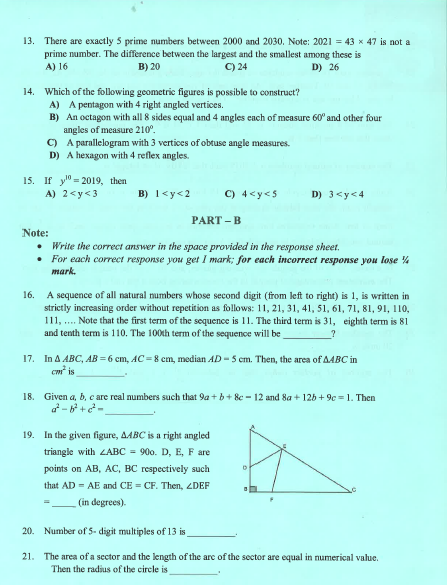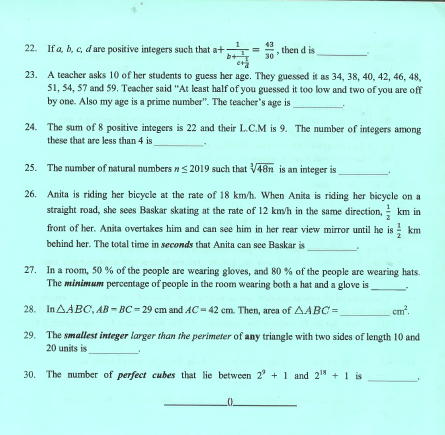## NMTC 2019 Sub Junior Level Answer Keys

NMTC 2019 Sub Junior Level
Ques NoAns KeyQues NoAns KeyQues NoAns KeyQues NoAns Key
1A9A1724253
2C10D18BONUS26600
3B11D19452730%
4D12D20692328420
5C13D2122960
6B14B2243056
7B15A2347
8D161100247

## NMTC 2019 Sub Junior Level Solutions

Part A: Instruction:

Only One of the choices A, B, C, D is correct. For each correct response, you get 1 mark. For each incorrect response, you lose 1/2 mark.

NMTC 2019 Question Papers With Solutions Sub Junior Level Ques No 1:

If 4921 x D = ABBBD, then the sum of the digits of ABBBD x D is ______.

Options:

A. 19

B. 20

C. 25

D. 26

Solution: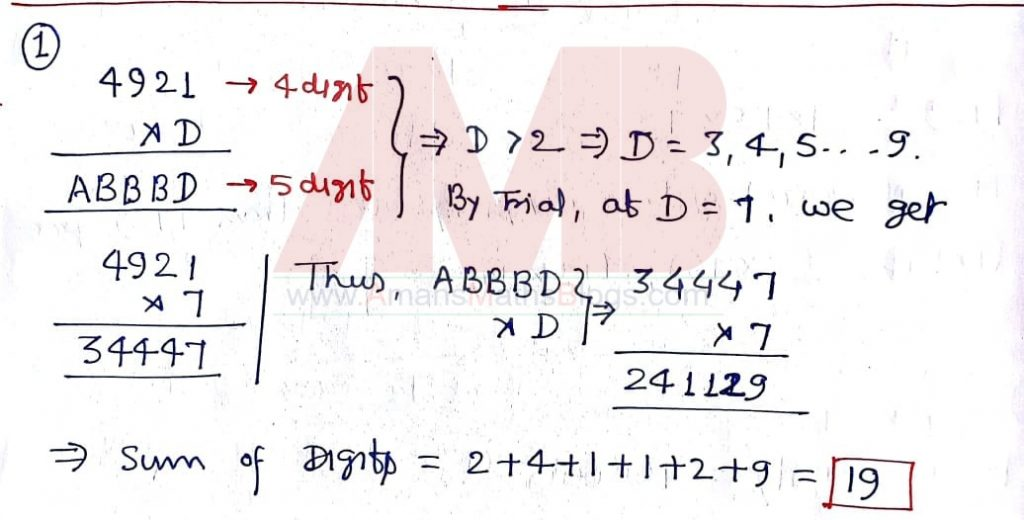NMTC 2019 Question Papers With Solutions Sub Junior Level Ques No 2:

What is the 2019th digit to the right of the decimal point, in the decimal representation of 5/28?

Options:

A. 2

B. 4

C. 8

D. 7

Solution:NMTC 2019 Question Papers With Solutions Sub Junior Level Ques No 3:

If X is a 1000 digit number, Y is the sum of its digits, Z is the sum of the digits of Y and W the sum of the digits of Z, then the maximum possible value of W is

Options:

A. 10

B. 11

C. 12

D. 22

Solution:NMTC 2019 Question Papers With Solutions Sub Junior Level Ques No 4:

Let X be the number 0.0000….001 which has 2019 zeroes after the decimal point. Then which of the following numbers is the greatest?

Options:

A. 10000 + x

B. 10000x

C. 10000/x

D. 1/x2

Solution: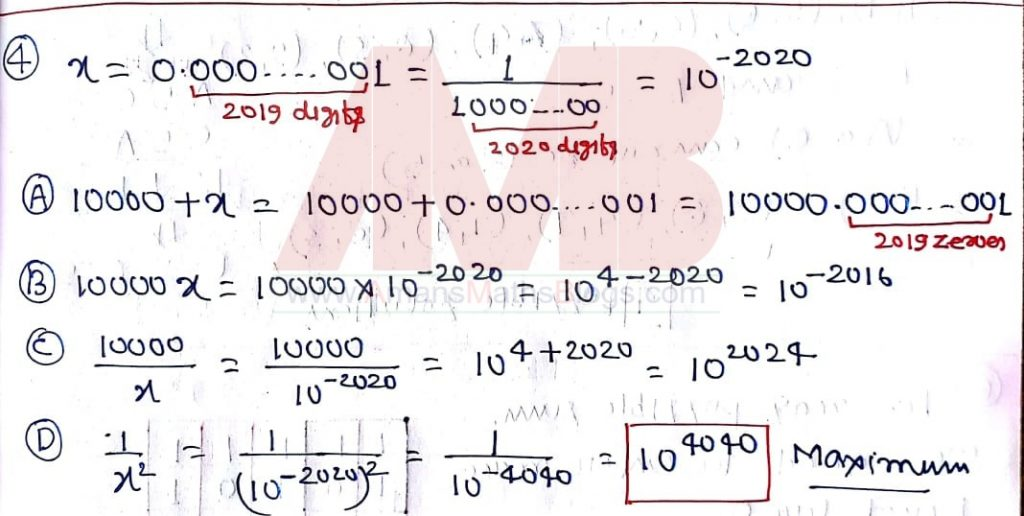NMTC 2019 Question Papers With Solutions Sub Junior Level Ques No 5:

Ifthen the number of possible values of A, B, C, D, E satisfying this equation where A, B, C, D, E are distinct digits is

Options:

A. 6

B. 5

C. 4

D. 3

Solution: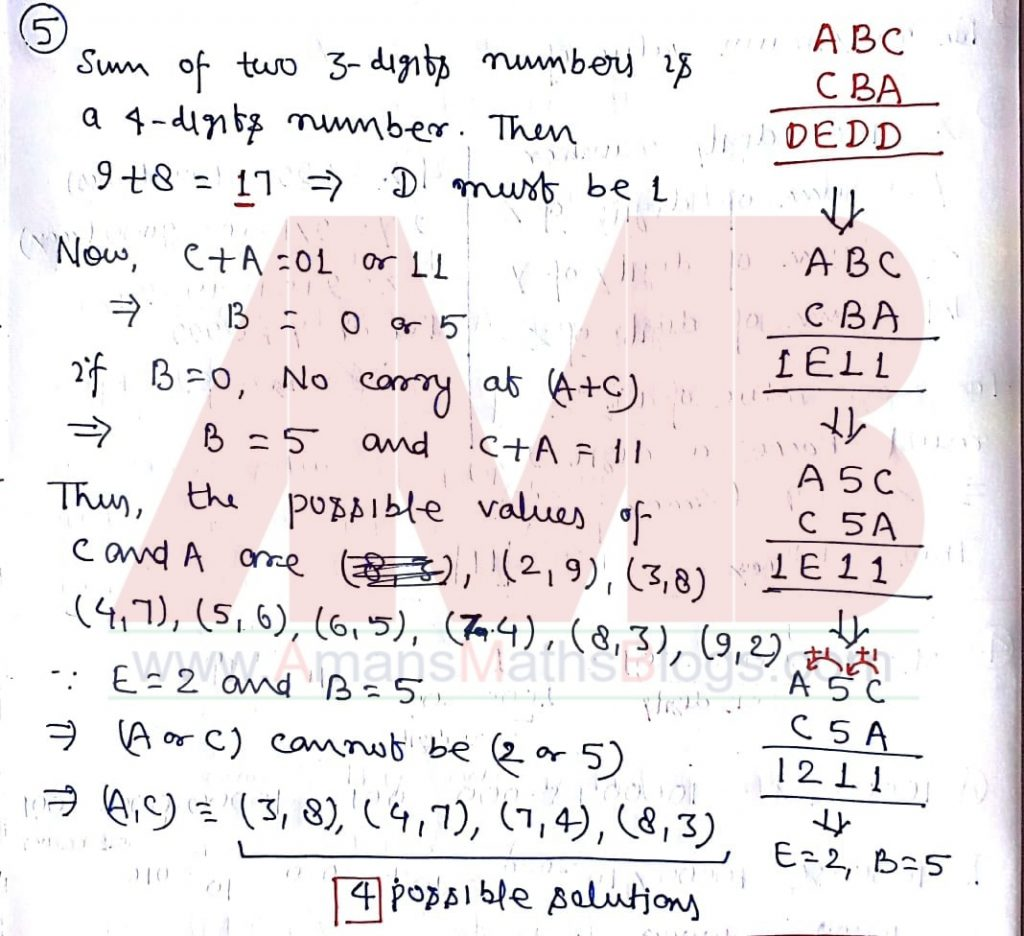NMTC 2019 Question Papers With Solutions Sub Junior Level Ques No 6:

In a 5 x 5 grid having 25 cells, Janani has to enter 0 or 1 in each cell such that each sub square grid of size 2 x 2 has exactly three equal numbers. What is the maximum possible sum of the numbers in all the 25 cells put together.

Options:

A. 23

B. 21

C. 19

D. 18

Solution:NMTC 2019 Question Papers With Solutions Sub Junior Level Ques No 7:

ABCD is a square. E is one fourth of the way from A to B and F is one fourth of the way from B to C. X is the center of the square. Side of the square is 8 cm. Then the area of the shaded regions in the figure in cm2 isOptions:

A. 14

B. 16

C. 18

D. 20

Solution:NMTC 2019 Question Papers With Solutions Sub Junior Level Ques No 8:

ABCD is a rectangle with E and F are midpoints of CD and AB respectively and G is the mid point of AF. The ratio of the area of ABCD to the area of AECG is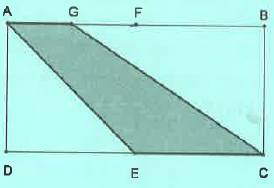Options:

A. 4 : 3

B. 3 : 2

C. 6 : 5

D. 8 : 3

Solution: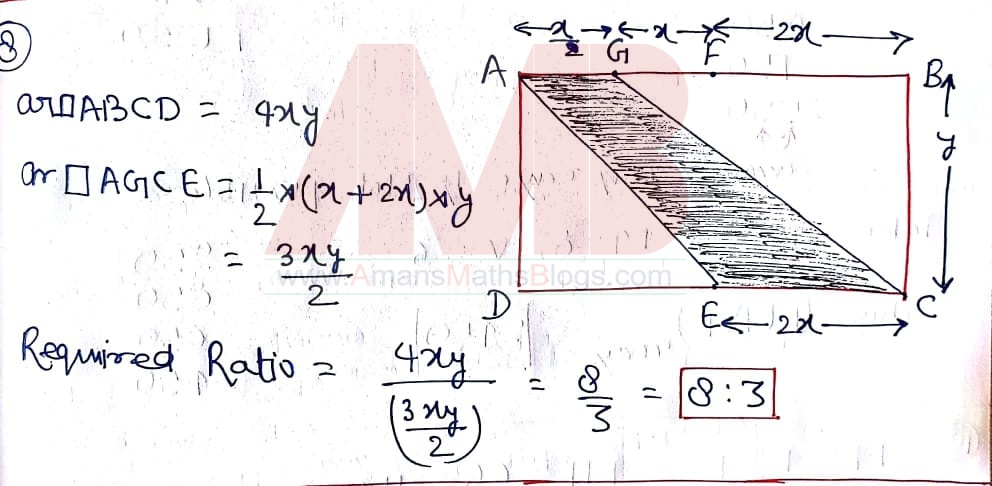NMTC 2019 Question Papers With Solutions Sub Junior Level Ques No 9:

If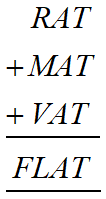and each alphabet represents a different digit, what is the maximum possible sum of the value of FLAT?

Options:

A. 2450

B. 2405

C. 2305

D. 2350

Solution: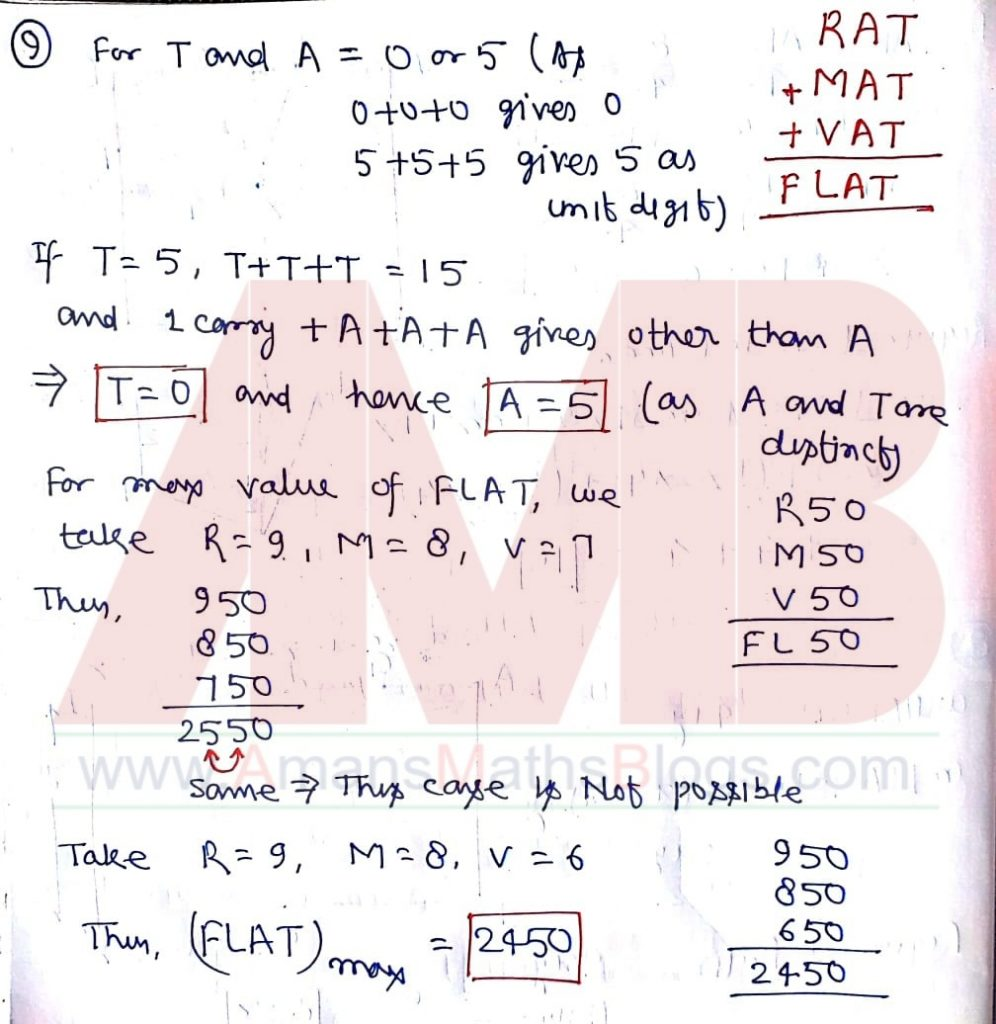NMTC 2019 Question Papers With Solutions Sub Junior Level Ques No 10:

How many positive integers smaller than 400 can you get as a sum of eleven consecutive positive integers?

Options:

A. 37

B. 35

C. 33

D. 31

Solution: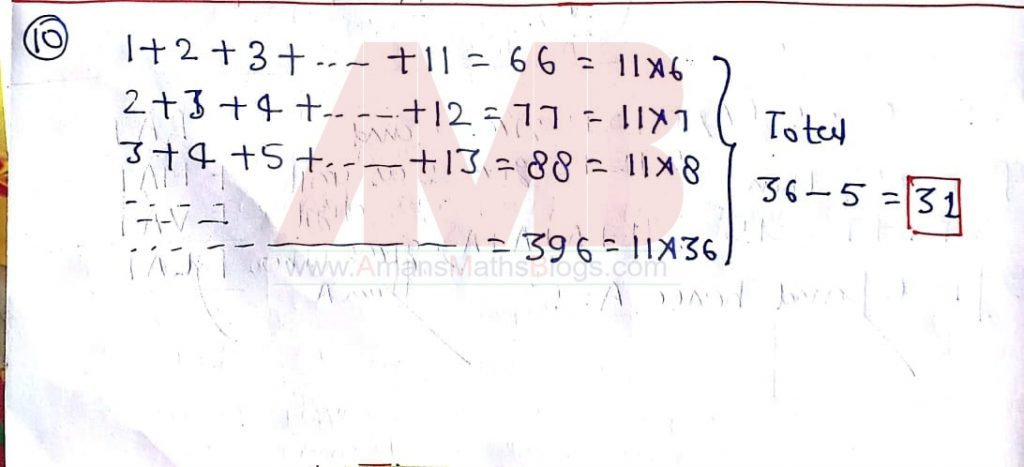NMTC 2019 Question Papers With Solutions Sub Junior Level Ques No 11:

Let x, y and z be positive real numbers and let x >= y >= y >= z so that x + y + z = 20.1. Which of the following statements is true?

Options:

A. Always xy < 99

B. Always xy > 1

C. Always xy75

D. Always yz49

Solution: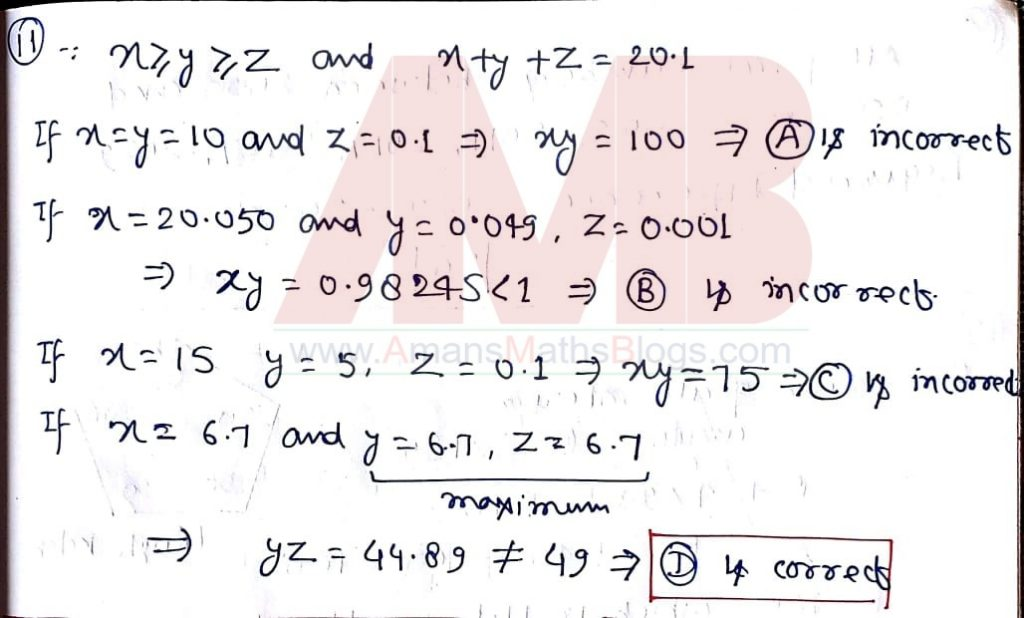NMTC 2019 Question Papers With Solutions Sub Junior Level Ques No 12:

A sequence {an} is generated by the rule, an = an-1 – an-2 for n >= 3. Given that a1 = 2 and a2 = 4, the sum of the first 2019 terms of the sequence is given by

Options:

A. 8

B. 2692

C. -2692

D. -8

Solution: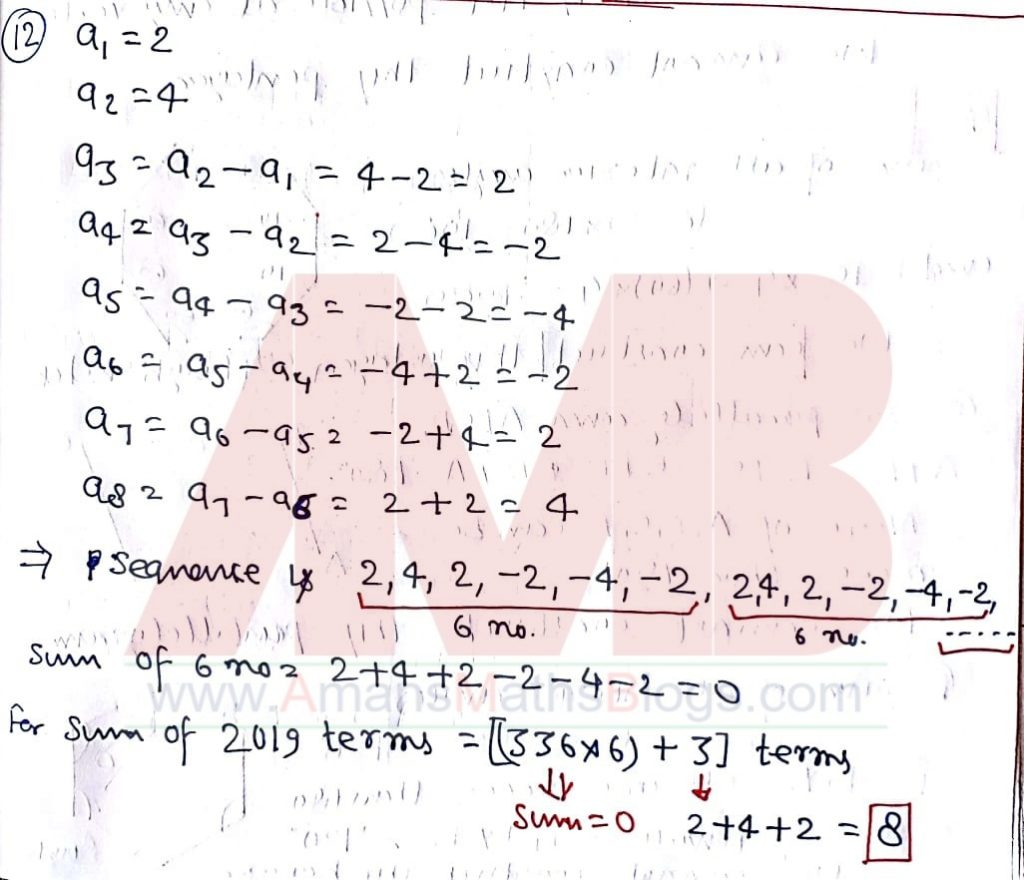NMTC 2019 Question Papers With Solutions Sub Junior Level Ques No 13:

There are exactly 5 prime numbers between 2000 and 2030. Note 2021 = 43 x 47 is not prime number. The difference between the largest and the smallest among these is

Options:

A. 16

B. 20

C. 24

D. 26

Solution:NMTC 2019 Question Papers With Solutions Sub Junior Level Ques No 14:

Which of the following geometric figure is possible to construct?

Options:

A. A pentagon with 4 right angled vertices.

B. An octagon with all 8 sides equal and 4 angles each of measure 60 degree and other four angles of measure 210 degree.

C. A parallelogram with 3 vertices of obtuse angle measure.

D. A hexagon with 4 reflex angles.

Solution: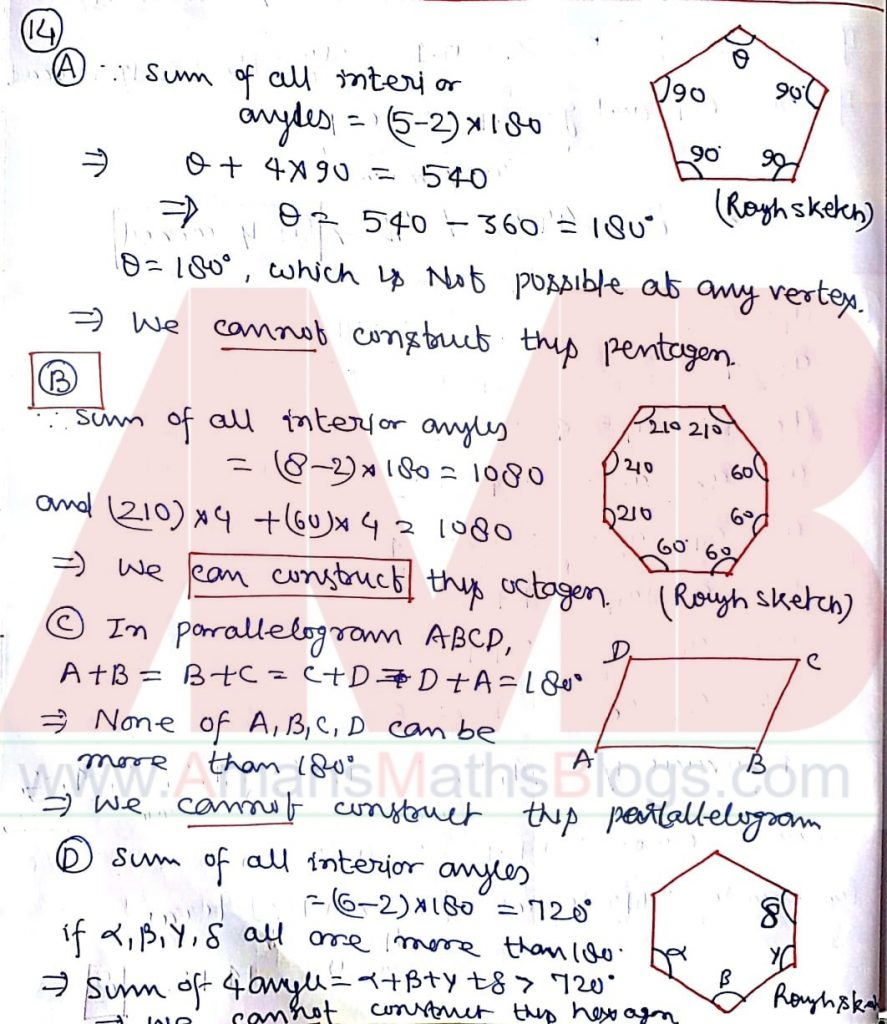NMTC 2019 Question Paper With Solutions Sub Junior Level Ques No 15:

If y10 = 2019, then

Options:

A. 2 < y < 3

B. 1 < y < 2

C. 4 < y < 5

D. 3 < y < 4

Solution: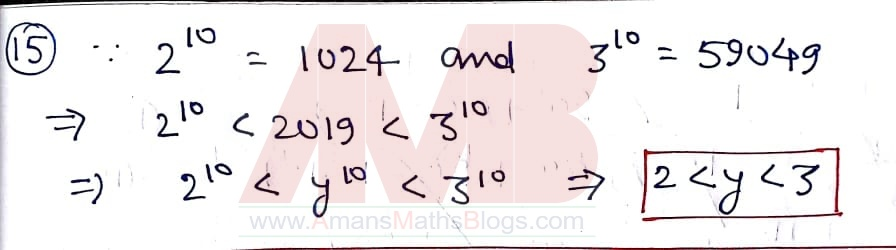Part B: Instruction:

Write the correct answer in the space provided in the responsive sheet. For each correct response, you get 1 mark. For each incorrect response, you lose 1/4 mark.

NMTC 2019 Question Paper With Solutions Sub Junior Level Ques No 16:

A sequence of all natural numbers whose second digit (from left to right) is 1, is written in strictly increasing order without repetition as follows 11, 21, 31, 41, 51, 61, 71, 81, 91, 111, … Note that the first term of the sequence is 11. The third term is 31. eighth term is 81 and tenth term is 110. The 100th term of the sequence will be ______ ?

Solution: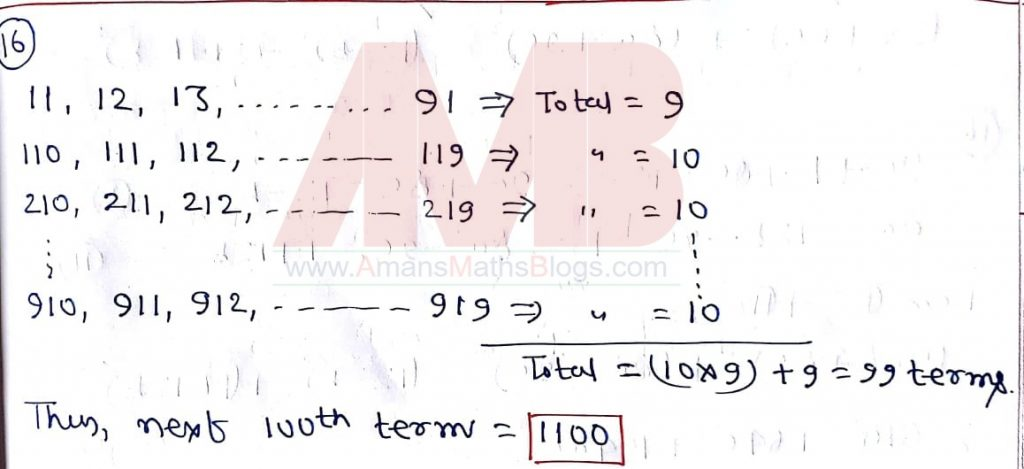NMTC 2019 Question Paper With Solutions Sub Junior Level Ques No 17:

In a triangle ABC, AB = 6 cm, AC = 8 cm median AD = 5 cm. Then, then area of the triangle ABC in cm2 is ______.

Solution: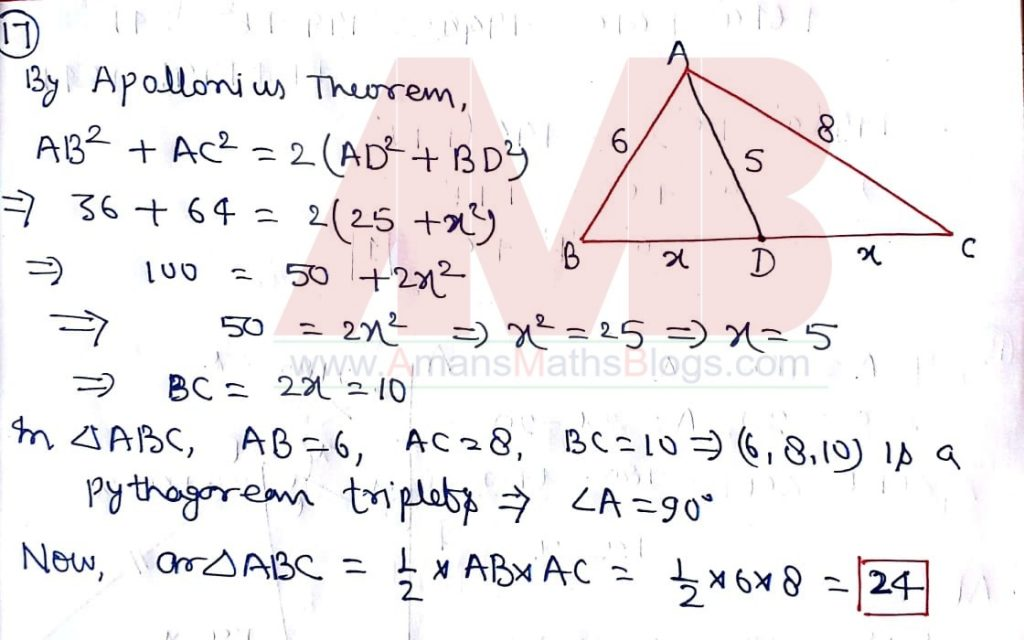NMTC 2019 Question Paper With Solutions Sub Junior Level Ques No 18:

Given a, b, c are real numbers such that 9a + b + 8c = 12 and 8a + 12b + 9c = 1. Then a2 – b2 + c2  is _____.

Solution: BONUS as given equations are incorrect. Correct equations are as below in solution.NMTC 2019 Question Paper With Solutions Sub Junior Level Ques No 19:

In the given figure, triangle ABC is a right angled triangle with angle ABC = 90 degree. D, E,F are points on AB, AC, BC respectively such that AD = AE and CE = CF. Then angle DEF is (in degree) ________.Solution: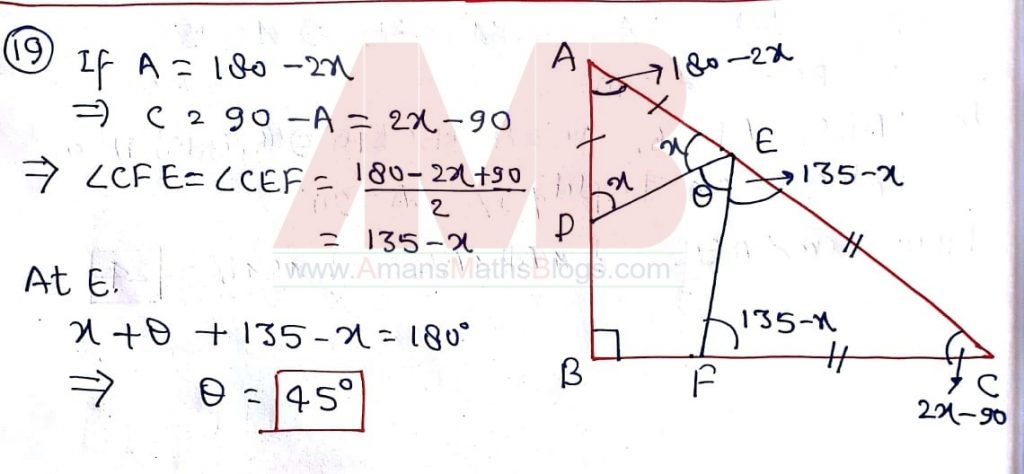NMTC 2019 Question Paper With Solutions Sub Junior Level Ques No 20:

Number of 5-digit multiple of 13 is _____.

Solution: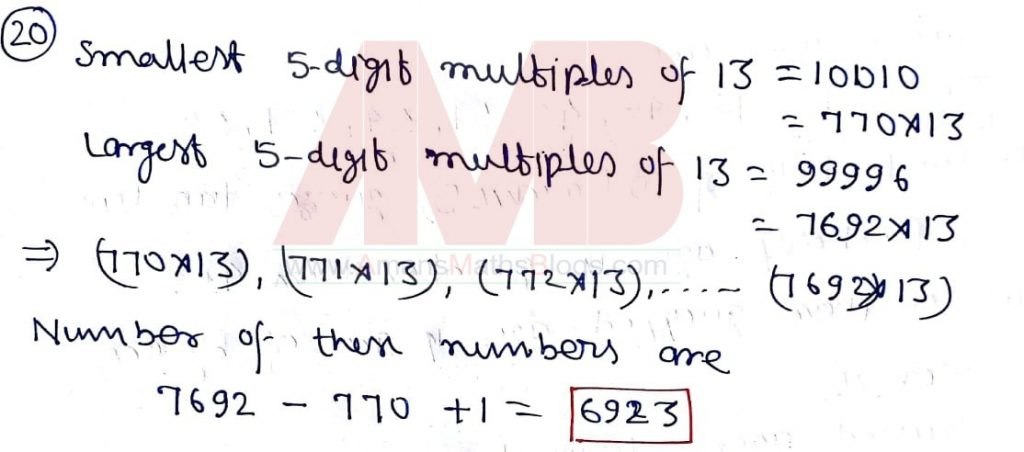NMTC 2019 Question Paper With Solutions Sub Junior Level Ques No 21:

The area of a sector and the length of the arc of the sector are equal in numerical value. Then the radius of the circle is _____.

Solution: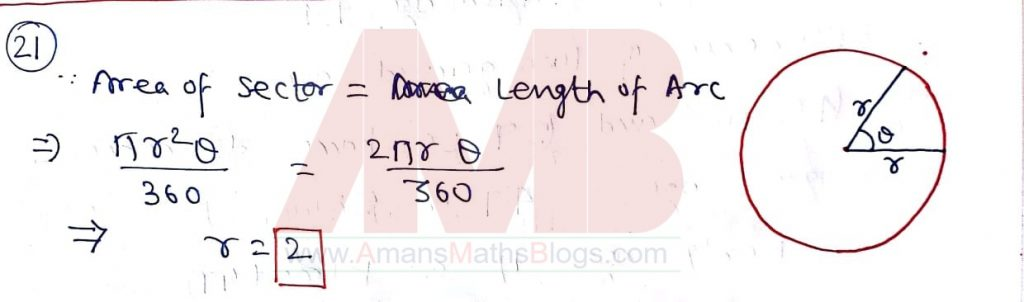NMTC 2019 Question Paper With Solutions Sub Junior Level Ques No 22:

if a, b, c, d are positive integers such that,then d is _______.

Solution: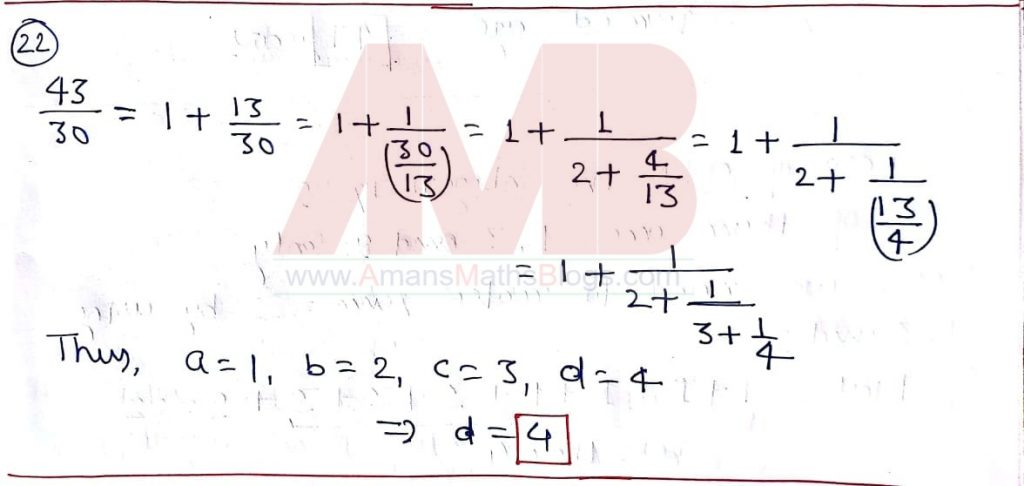NMTC 2019 Question Paper With Solutions Sub Junior Level Ques No 23:

A teachers asks 10 of her students to guess her age. They guessed it as 34, 38, 40, 42, 46, 48, 51, 54, 57 and 59. Teacher’s said, “At least half of you guessed it too low and two of you are off by one. Also my age is a prime number”. The teacher’s age is ______.

Solution:NMTC 2019 Question Paper With Solutions Sub Junior Level Ques No 24:

The sum of 8 positive integers is 22 and their LCM is 9. The number of integers among these that are less 4 is _______.

Solution: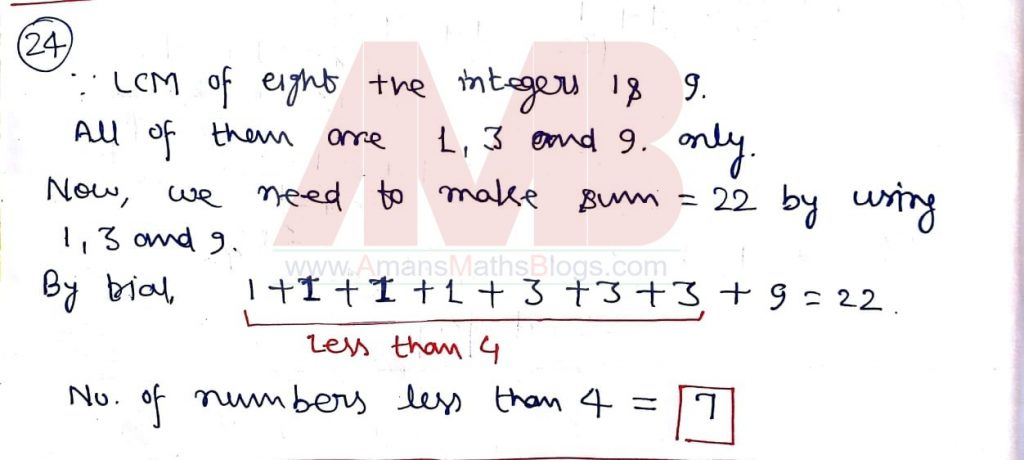NMTC 2019 Question Paper With Solutions Sub Junior Level Ques No 25:

The number of natural numbers n <= 2019 such that ∛(48n) is an integer is ________ .

Solution: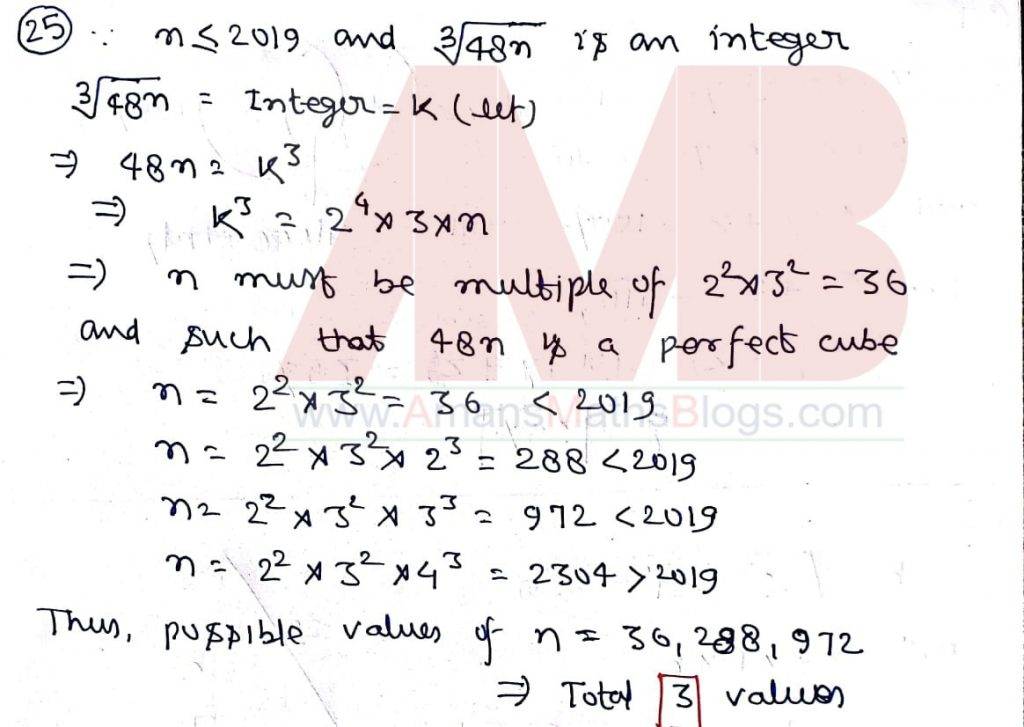NMTC 2019 Question Paper With Solutions Sub Junior Level Ques No 26:

Anita is riding her bicycle at the rate of 18 km/h. When Anita is riding her bicycle on a straight road, she sees Baskar skating at the rate of 12 km/h in the same direction, 1/2 km in front of her. Anita overtakes him and can see him in her rear view mirror untill he is 1/2 km behind her. The total time in seconds that Anita can see Baskar is ______.

Solution: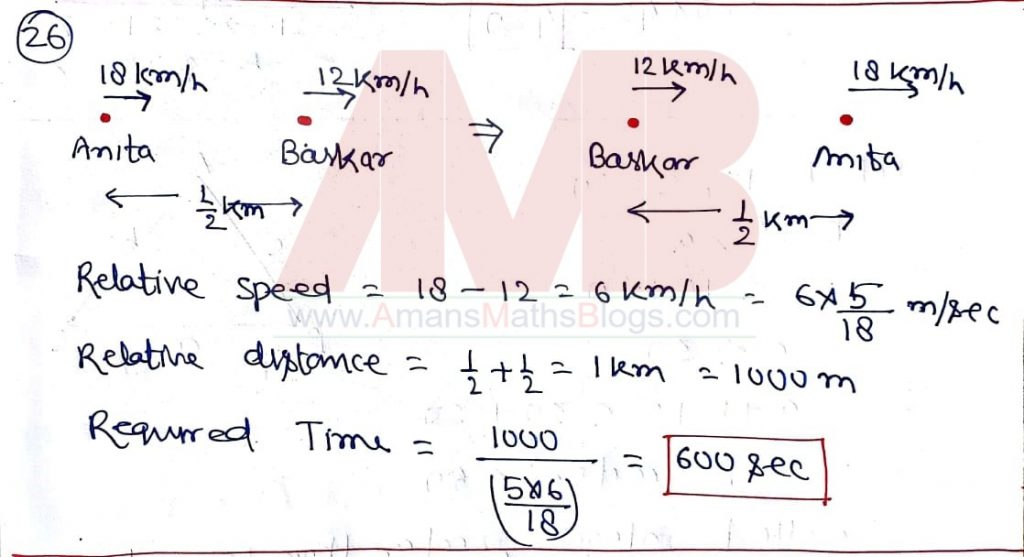NMTC 2019 Question Paper With Solutions Sub Junior Level Ques No 27:

In a room, 50% of the people are wearing gloves and 80% of the people are wearing hats. The minimum percentage of the people in the room wearing both a hat and a glove is ______.

Solution:NMTC 2019 Question Paper With Solutions Sub Junior Level Ques No 28:

In a triangle ABC, AB = BC = 29 cm and AC = 42 cm. Then the area of the triangle ABC = ____ cm2.

Solution:NMTC 2019 Question Paper With Solutions Sub Junior Level Ques No 29:

The smallest integer larger than the perimeter of any triangle with two sides of length 10 and 20 units is _______.

Solution:NMTC 2019 Question Paper With Solutions Sub Junior Level Ques No 30:

The number of perfect cubes that lie between 29 + 1 and 218 + 1 is _______.

Solution:

Get Online Maths Tuition by AMBiPi for Class 8 to 12